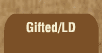Mathematics and Dyslexia

Tips for Learning the Times Tables

Tools for the Times Tables & Workbook

Home > Math > times tables practice > Nine Times Tables(all, scrambled)

## The Nines Times Tables

The digits to numbers that you can divide by nine always add up to nine.

You may only have to do this page once or twice! If not, you might want to go back and practice the smaller groups that feature the ones you still aren't quick with.

1. 9 x 3=
2. 9 x 5=
3. 1 x 9=
4. 9 x 9=
5. 9 x 7=
6. 10 x 9=
7. 6 x 9=
8. 9 x 4=
9. 8 x 9=
10. 9 x 2=
11. 9 x 10=
12. 0 x 9=
13. 7 x 9=
14. 9 x 8=
15. 9 x 9=